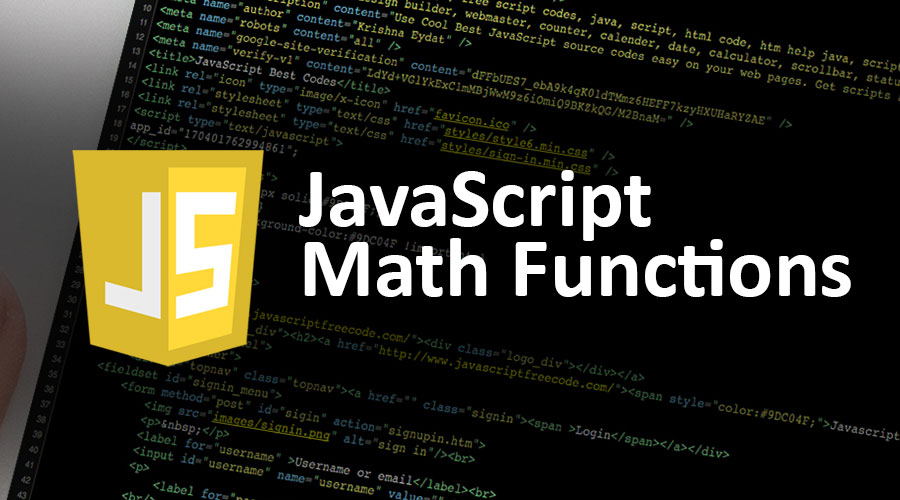# JavaScript Math Functions## Introduction to JavaScript Math Functions

The JavaScript Math is a built-in object that provides properties and methods for mathematical constants and functions to execute mathematical operations. It is not a function object, not a constructor. You can call the Math as an object without creating it because the properties and methods of Math are static.

### JavaScript Math Functions

The Math Functions consist of methods and properties. Following is the list of methods used with the Math object:

#### 1)Math.round()

This method provides the value of the given Number to a rounded integer. It can be written as:
Math.round(x), where x is a number.

#### 2)Math.pow()

It provides the value of x to the power of y. It can be written as:
Math.pow(x, y), where x is a base number and y is an exponent to the given base.

#### 3)Math.sqrt()

It gives the square root of a given integer. It can be written as:
Math.sqrt(x), where x is a number.

#### 4)Math.abs()

It provides the absolute i.e. positive value of a number. It can be written as:
Math.abs(x); where x is a number.

#### 5)Math.ceil()

It gives a smaller number, which is greater or equal to the given integer. It can be written as:
Math.ceil(x); where x is a number

#### 6)Math.floor()

It gives a larger number, which is lesser or equal to the given integer. It can be written as:
Math.floor(x); where x is a number.

#### 7)Math.sin()

It provides a sine of the given number. It can be written as:
Math.sin(x); where x is a number.

#### 8)Math.cos()

It provides cosine of the given number. It can be written as:
Math.cos(x); where x is a number

#### 9)Math.min() and Math.max()

The min() method is used to display the lowest value of the given arguments. It can be written as:
Math.min(val1, val2………valn); where val1, val2………valn are numbers.

The max() method is used to display the highest value of the given arguments. It can be written as:
Math.max(val1, val2………valn); where val1, val2………valn are numbers.

#### 10)Math.random()

It provides a random number between 0 and 1. It can be written as:
Math.random();

#### 11)Math.acos()

It provides an arccosine of an integer. It can be written as:
Math.acos(x); where x is a number.

#### 12)Math.asin()

It provides arcsine of an integer. It can be written as:
Math.asin(x); where x is a number.

### Examples

Let us see few examples for the above some methods of JavaScript Math Functions:

Output

5.6

Output

8

Output

5

• #### Math.sin()

Output

First Value: -0.977530117665097

Second Value: 0.8939966636005579

Third Value: 1

• #### Math.cos()

Output

First Value: -0.4480736161291702

Second Value: 0.5403023058681398

Third Value: 1

• #### Math.min() and Math.max()

``` Minimum Value: ```

``` Maximum Value: ```

Output

Minimum Value:

14

Maximum Value:

90

• #### Math.random()

Output

First Value: 0.6817374601673007

Second Value: 0.5478539257364348

Third Value: 0.4483563080449702

• #### Math.acos()

Output

First Value: 3.141592653589793

Second Value: 1.5707963267948966

Third Value: NaN

Fourth Value: NaN

• #### Math.asin()

Output

First Value: -1.5707963267948966

Second Value: 0

Third Value: NaN

Fourth Value: NaN

### Here is the list of properties used with Math object:

1)E- It specifies Euler’s number.

Examples of E-

Output: The Value is 2.718281828459045.

2)PI- It provides PI value.

Examples of PI-

Output: The Value is 3.141592653589793.

3)SQRT2- It specifies the square root of 2.

Examples of SQRT2-

Output: The Value is 1.4142135623730951.

4)SQRT1_2- It specifies the square root of 1/2.

Examples of SQRT1_2-

Output: The Value is 0.7071067811865476.

5)LN2- It specifies the natural logarithm of 2.

Examples of LN2-

Output: The Value is :0.6931471805599453.

6)LN10- It specifies the natural logarithm of 10.

Examples of LN10-

Output: The Value is 2.302585092994046.

7)LOG2E- It specifies the BASE 2 logarithm of E.

Examples of LOG2E-

Output: The Value is 1.4426950408889634.

8)LOG10E- It specifies the BASE 10 logarithm of E.

Examples of LOG10E-

Output: The Value is 0.4342944819032518.

### Conclusion

As we discussed, you cannot consider the Math object as the constructor. It provides built-in properties and methods for performing mathematical tasks on numbers. To make use of the Math object, extend the Math object directly instead of using the prototype.

### Recommended Articles

This has been a guide to JavaScript Math Functions. Here we discuss the introduction, methods, and properties of the Math Functions with their corresponding examples. You can also go through our other suggested articles to learn more –

1. How JavaScript Works
2. JavaScript String Functions
3. How to Install JavaScript
4. Careers in JavaScript

The post JavaScript Math Functions appeared first on EDUCBA.

This post first appeared on Best Online Training & Video Courses | EduCBA, please read the originial post: here